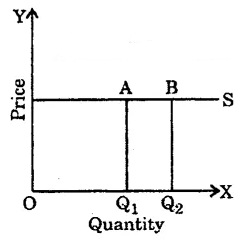• #### 1.  The figure given below shows:

• Extension in Supply
• Contraction in Supply
• Elasticity of supply
• Elasticity of demand
• #### 2.  The quantity of a goods which the seller is ready to sell in the market at fixed price and time is called ?

• Supply
• Demand
• Elasticity of supply
• Elasticity of Demand
• #### 3.  Supply is associated with:

• A Time Period
• Price
• Both (a) and (b)
• None of the above
• #### 4.  Determinating factor of supply of goods is:

• Price of Goods
• Price of Related Goods
• Price of Factor of Production
• All the above
• #### 5.  Which of the following statement is true ?

• Price and quantity have direct relationship
• Supply curve rises from left to right
• Supply is affected by many factors
• All the above
• #### 6.  Which of the following function shows the laws of supply ?

• S = f(P)
• S = f(a/p)
• S = f(Q)
• None of the above
• #### 7.  Following figure shows :

• Perfectly Elastic Supply
• Perfectly Inelastic Supply
• Elastic Supply
• Inelastic Supply
• #### 8.  Which of the following is correct ?

• Perfectly Elastic Supply e
• High Elastic Supply e
• Perfectly Inelastic Supply e
• All the above
• #### 9.  Following figure shows :• Perfectly Elastic Supply
• Perfectly Inelastic Supply

• Less Elastic Supply
• Unit Elastic Supply
• #### 10.  If the price of goods rises by 60% but supply increases by only 5%, the supply of goods will be:

• Highly Elastic
• Elastic
• Inelastic
• Perfectly Inelastic
Report Question
Practice More On Production and Costs
access_time